# Understanding FFT for Beginners

Posted by Valery Zaichenko on

OK, my take on spectrum measurements :)

This kind of picture is a result of Fast Fourier Transform (FFT) - mathematical transformation of the input signal.
Fourier says - every input signal (whatever form it is) can be represented as a combination of sine waves.
Each "stick" on the spectrum diagram represents a single sine wave of particular frequency and amplitude.

Thus, every component is characterized by Amplitude (V) and Frequency (F).

Now - some explanation for each picture: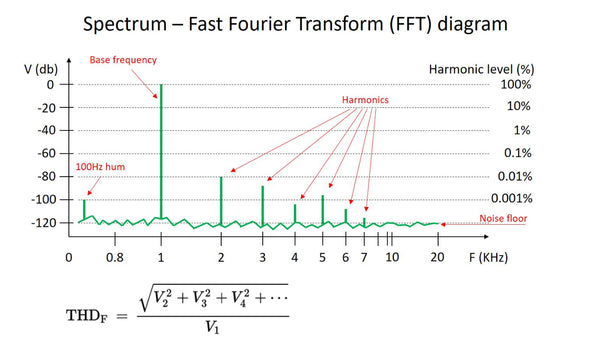1) Typical FFT diagram. Base frequency component represents the test signal (1KHz sine wave) - set to 0db. A small (sometimes not so small :P) stick on the left appears because of some PSU ripple (100Hz in Europe, 120Hz in US).
All the sticks on the right - harmonics of the base signal (F*2, F*3, F*4 and so on). It is noted that all the even components (2, 4, 6, etc.) are less audible (and more pleasing to some listeners) than the odd components (3, 5, 7, etc.).
Knowing the amplitude of each component, you can easily calculate the Total Harmonic Distortion (THD, db or %) at particular base frequency - see the formula at the bottom of the picture (measurement system makes this calculation automatically for you).
You can measure distortion at any base frequency - normally, it's 1KHz and 10KHz or 20KHz (if the sound card is used for measurements, for showing 20KHz harmonics adequately, it must be a good wide-band low-distortion sound card).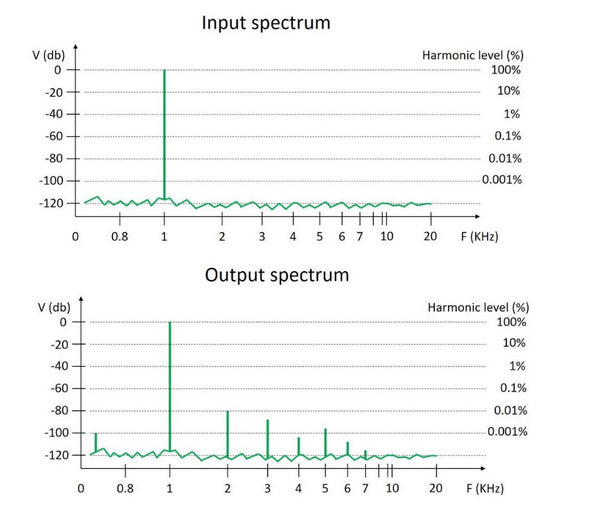2) This is just to illustrate that an amplifier adds some components that are not in the input signal - this is what we hear as distortion. The output spectrum shown is not bad - 2-nd harmonic dominance, some 3-rd, all the rest are roughly at -100db and below.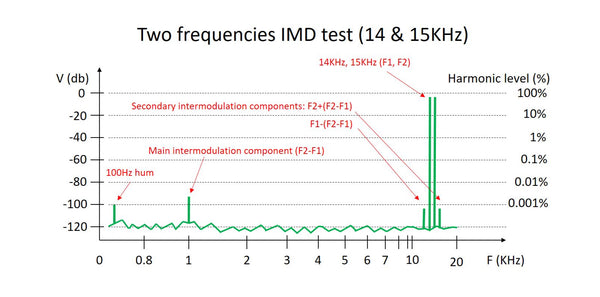3) Another very important measurement - Inter-Modulation Distortion (IMD).
You send a mixture of two sine waves to the input - for example, 14KHz and 15KHz (in this case each of them we normally set to -6db, then level of the mix will appear at 0db, as they sum up).
Passing through the amplifier, they inter-modulate each other, producing the addition (F2+F1) and subtraction (F2-F1) frequency components. I our case, (F2+F1) = 15 + 14 = 29KHz is not that interesting, but (F2-F1) = 15 - 14 = 1KHz is something we are really interested in. It's placed in the very audible frequency range and it was completely missing in the input signal - so we don't want to have it at the output.
In addition to that, 3-rd order (as well as any higher-order) inter-modulation occurs, so we see F2+(F2-F1) and F1-(F2-F1) - and so on - components close to our F1 and F2 base signals' sticks.
The real music signal consists of many components at the input - just imagine - they all inter-modulate each other and all the products of this inter-modulation inter-modulate each-other and so on. Horror. Lots of new stuff, completely out of sync with the input signal. Good amplifier keeps the number and the level of these components relatively low.
Click on the link to see an example of an exceptionally low live-measured IMD: Vertical CFA + NS-OPS IMD.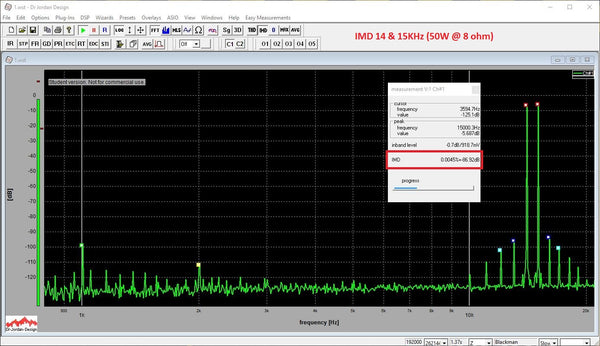4) Example of IMD live measurement. You can clearly see 1KHz component, 3-rd order components on the right, and - by the way – the other small component at 2KHz. Good amplifier.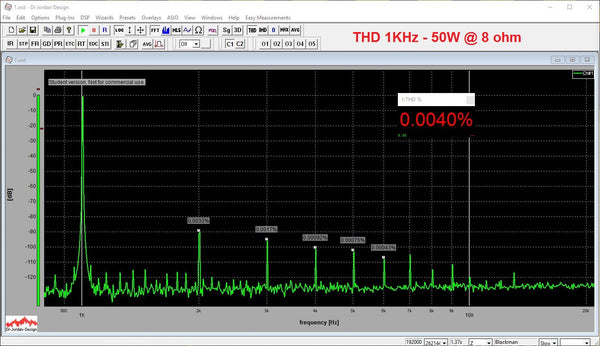5) Example of THD live measurement. Also a good one - 2-nd harmonic is dominant, 3-rd is half of it in amplitude (-6db), all the rest are below -100db level. Note some 100Hz recurring components between 1KHz and 2KHz - a little bit of PSU ripple, very low level though. Also, note a small glitch at around 13KHz - that's a measurement system artifact.

These two spectrums above are taken on VHex+BT (Bi-Turbo double-bootstrap version).

This kind of diagrams (especially if they are available at different frequencies and amplitudes) allow seeing the amplifier's character - assessing not only harmonics' amplitudes but also those amplitudes distribution (profile) and ratios between them.

I hope this is enough as a first introduction to FFT. Let me know is you will have questions ;)

Cheers,
Valery